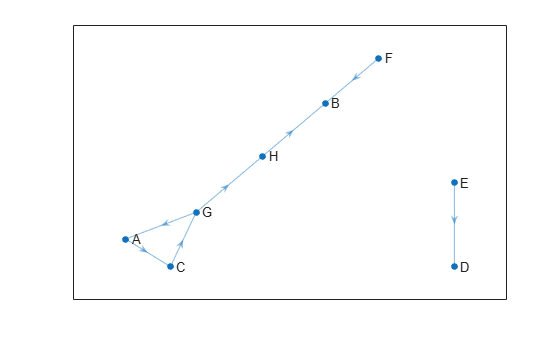Since R2023a

## Syntax

``linkIDS = findlink(graph,statePairs)``

## Description

example

````linkIDS = findlink(graph,statePairs)` finds the link IDs `linkIDS` of the state pairs `statePairs`, if they exist in the `navGraph` object `graph`.```

## Examples

collapse all

`load navGraphData.mat`

```stateTable = table(data.states,data.names,data.numLanes, ... VariableNames=["StateVector","Name","Lanes"]); linkTable = table(data.links,data.linkWt,data.curvature, ... VariableNames=["EndStates","Weight","Curvature"]);```

Create a `navGraph` object from the state and link tables.

`graphObj = navGraph(stateTable,linkTable);`

Create a deep copy of the `navGraph` object.

`graph2 = copy(graphObj)`
```graph2 = navGraph with properties: States: [8x3 table] Links: [7x3 table] LinkWeightFcn: @nav.algs.distanceEuclidean ```

Visualize the `navGraph` object.

`show(graphObj)`Find the link IDs of two state pairs. The function returns the link ID for the state pair `["G","A"]`. However, it returns `0` as the link ID for the state pair `["C","D"]` as the link does not exist in the `navGraph` object.

`linkIDS = findlink(navGraphObj,["G","A"; "C","D"])`
```linkIDS = 2×1 5 0 ```

## Input Arguments

collapse all

Graph object, specified as a `navGraph` object.

State pairs, specified as pairs of state names or pairs of state IDs.

Specify the pairs of state names as an N-by-2 string array or N-by-2 cell array of character vectors. Specify the pairs of state IDs as an N-by-2 matrix of positive integers. N is the number of state pairs specified.

Data Types: `single` | `double` | `cell` | `char` | `string`

## Output Arguments

collapse all

Link IDs, returned as a column vector of positive integers. When the function does not find a specified state pair, the value for the corresponding element in `linkIDS` is `0`.

Data Types: `double`

## Version History

Introduced in R2023a